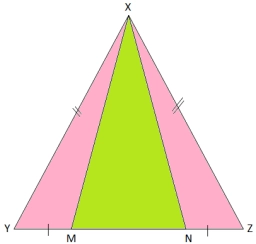# Equilateral 80573

The field has the shape of an equilateral triangle. Calculate its content if you know that the side is 280 meters long.

S =  339.482 a

### Step-by-step explanation:Did you find an error or inaccuracy? Feel free to write us. Thank you!

Tips for related online calculators
Need help calculating sum, simplifying, or multiplying fractions? Try our fraction calculator.
The Pythagorean theorem is the base for the right triangle calculator.
Calculation of an equilateral triangle.